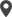# Chapter 4 - Odoo 15 Development Book

## Onchange with Compute Method

Onchange and compute logic are mostly used techniques in odoo to achieve some kind of business logic. A Computed field is a field for which the value is computed using values of other fields. The method used for computing the value of a computed field is commonly referred to as compute methods, whereas methods triggered with field value change are onchange methods.

Let's discuss both cases with the help of an example. Consider a business model to manage Rental orders, where the total rent amount is calculated from the duration and price for the unit duration. For this purpose, let us define a monetary field total_rent as in the following code, which is a computed field where the value of this field is computed using the value of another field or more than one field.

``````from odoo import models, fields
class VehicleRental(models.Model):
_name = "vehicle.rental"
_description = "Vehicle Rental"
hour_rate = fields.Monetary(string="Hour Rate",)
hours = fields.Integer(string="Hours")
total_rent = fields.Monetary(string='Total Rent',
compute='_compute_total_rent')
def _compute_total_rent(self):
for record in self:
record.total_rent = record.hour_rate*record.hours``````

Usually, the value of computed fields is dependent on other fields. Therefore, it is better to include the dependencies in the compute method. The ORM expects the developer to specify those dependencies with the decorator depends() on the method. ORM uses the given dependencies to trigger the compute method whenever any change occurs in the dependent fields.

``````from odoo import models, fields, api
class VehicleRental(models.Model):
_name = "vehicle.rental"
_description = "Vehicle Rental"
hour_rate = fields.Monetary(string="Hour Rate",)
hours = fields.Integer(string="Hours")
total_rent = fields.Monetary(string='Total Rent',
compute='_compute_total_rent')
@api.depends('hour_rate', 'hours')
def _compute_total_rent(self):
for record in self:
record.total_rent = record.hour_rate*record.hours``````

The onchange mechanism in Odoo enables the feature to modify or update the value of a field if any update is done on the other fields. Furthermore, the onchange will be triggered when one of the given fields is modified in the form view, that is, they are only triggered in the form view. In the given example the Reference for the rental order is achieved from a method. The onchange() decorator enables the feature to trigger the onchange method whenever the vehicle_id field in the form changes its value.

``````from odoo import models, fields, api
class VehicleRental(models.Model):
_name = "vehicle.rental"
_description = "Vehicle Rental"
name = fields.Char(string="Ref.")
vehicle_id = fields.Many2one('fleet.vehicle', string="Vehicle")
@api.onchange('vehicle_id')
def _onchange_vehicle(self):
self.name = "Rental Order for %s" % self.vehicle_id.name``````

### Model Based on SQL View

In most cases, we create a model class with fields defined.This will be mapped to database tables by Odoo.There may be some cases where we need to aggregate data from several models to a single table.This may be useful for making dashboards or generating reports. Making use of the postgresql database engine in odoo it is possible to create a read only model backend by postgresql view rather than a database table.

Lets discuss it with the help of an example.Consider a model student.student which stores all the student details of an educational organization.

1. Create a new model with _auto attribute set to False.

``````class StudentLeaveStatistics(models.Model):
_name = 'student.leave.statistics'
_auto = False``````

2. Define fields for the model and set the readonly attribute as False so that the Views do not enable modifications that you will not be able to save, since PostgreSQL Views are read-only.

``````student_id = fields.Many2one('student.student', string="Student",

3. Next step is to define init() method to create the view.This method is responsible for creating table when auto attribute is set to False. Otherwise, this does nothing. The view creation query must create a view with column names that match the field names of the Model.

``````def init(self):
tools.drop_view_if_exists(self._cr, 'student_leave_statistics')
self._cr.execute("""
create or replace view student_leave_statistics as (
select
min(sl.id) as id,
sl.student_id as student_id,
count(sl.id) as leave_count,
from
student_leave as sl
join
student_student as s ON s.id = sl.student_id
where
sl.state = 'done'
)""")``````

4. You can now define Views for the new model. A pivot view is especially useful to explore the dataCalicut

Cybrosys Technologies Pvt. Ltd.
Neospace, Kinfra Techno Park
Kakkancherry, Calicut
Kerala, India - 673635London

Cybrosys Limited
Alpha House,
100 Borough High Street, London,
SE1 1LB, United KingdomKochi

Cybrosys Technologies Pvt. Ltd.
1st Floor, Thapasya Building,
Kochi, India - 682030.Bangalore

Cybrosys Techno Solutions
The Estate, 8th Floor,